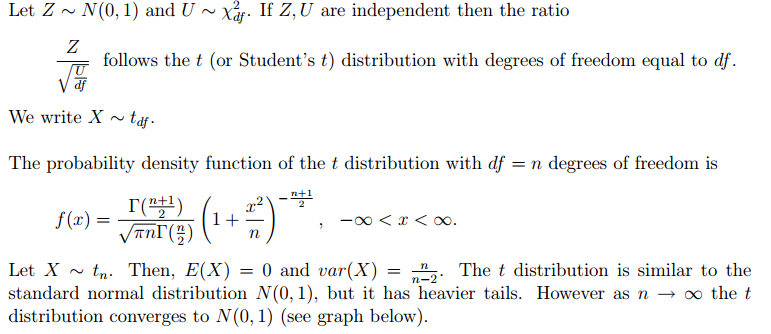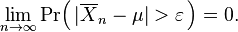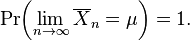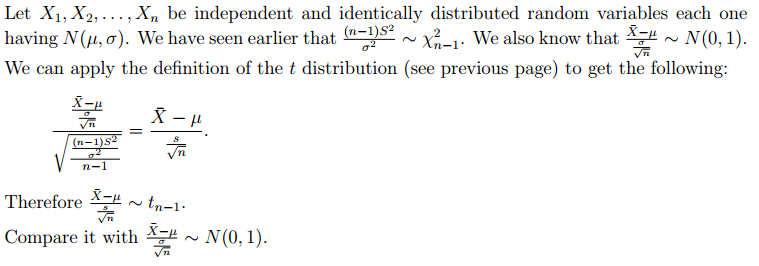if Z is Normal and U has Chi squared distribution.

According to T1 Z^2 has Chi squared distribution with 1 df.

According to T3:has an F distribution of F(1,n).

Proof:

if x is normal, According to T1, Z^2 has Chi squared distribution with 1 df.

\frac{(x-\mu)^2}{\sigma^2/n}has chi squared distribution because x-mu is normal.

It can be proven that\frac{(n-1)S^2}{\sigma^2}\sim \chi^2_{n-1}

Therefore\frac{ (x-\mu)^2}}{\frac{S^2}{n}}=\frac{ \frac{(x-\mu)^2}{1/n}}{{S^2}}=\frac{ \frac{(x-\mu)^2}{\sigma^2/n}}{(\frac{S^2}{\sigma^2})}=\frac{ \frac{(x-\mu)^2}{\sigma^2/n}}{(\frac{(n-1)S^2}{\sigma^2})/(n-1)}\sim F_{(1,n-1)}

=============================================================

# Below we show thathas a  t^2 distribution by proving thathas a t distribution with n-1 df.

\frac{ (x-\mu)^2}{\frac{S^2}{n}}

—————————————–

# Definition# Laws of large numbers say:

Weak:Strong:# We know:

http://mathworld.wolfram.com/CentralLimitTheorem.html# or

http://www.ams.org/bookstore/pspdf/gsm-80-prev.pdf\lim_{n->\infty}(a\leq \frac{ \bar{W}-\mu }{\frac{\sigma}{\sqrt{n}} }\leq b)=\frac{1}{\sqrt{2\pi}} \int_{a}^{b} e^{\frac{-z^2}{2}}d_{z}

# We know

—————————————————————————————-The proof involves addition of two Chi squared pdfs which leads to either convolution:

http://www.dartmouth.edu/~chance/teaching_aids/books_articles/probability_book/Chapter7.pdf

or use of method of moments

Theorem 19.  http://digitalcommons.usu.edu/gradreports/14/   the best of proofs.

# Therefore:As shown above, The numerator is Normal based on central limit theorem, and the denominator is chi. therefore it is t.

===========================================================

Theorem 20.  http://digitalcommons.usu.edu/gradreports/14/   the best collection of proofs.